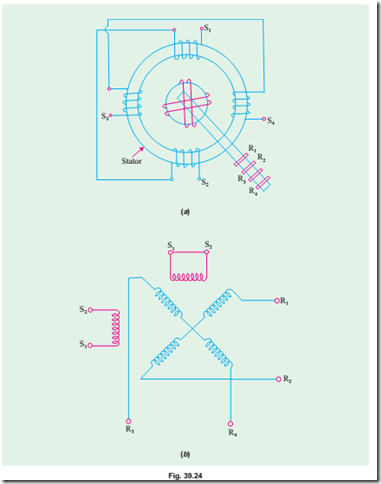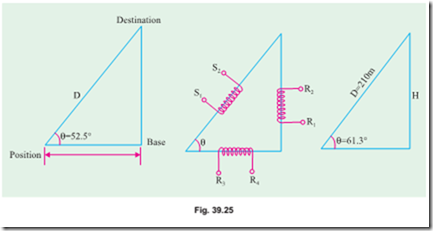# Special machines:The Resolver

##### The Resolver

In many ways, it is similar to a synchro but differs from it in the following respects : (i) Electrical displacement between stator windings is 90º and not 120º (ii) It has two stator windings and two rotor windings (Fig. 39.24) (iii) Its input can be either to the stator or to the rotor (iv) They are usually not used as followers because their output voltage is put to further use.###### (a) Construction

The main constructional features and the symbol for a resolver are shown in Fig. 39.24. There are two stator windings which are wound 90º apart. In most applications, only one stator winding is used, the other being short-circuited. The two rotor winding connections are brought out through slip rings and brushes.

###### (b) Applications

Resolvers find many applications in navigation and height determination as shown in Fig. 39.25

(a) nd (c) where Fig. 39.25 (b) provides the key.

As shown in Fig. 39.25 (a), the purpose is to determine the distance D to the destination. Suppose the range R to a base station as found by a radar ranging device is 369 km. The angle q is also determined directly. If the amplifier scale is 4.5 V per 100 km, the range would be represented by 369 ´ (4.5 / 100) = 16.6 V. Further suppose that angle q is found to be 52.5º. Now, set the resolver at 52.5º and apply 16.6 V to rotor terminals R3 R4. The voltage which appears at terminals S1 S2 represents D. If we assume K = stator turns / rotor turns = 1, the voltage available at S1 S2 will be = 16.6 / cos 52.5º = 16.6 / 0.6088 = 27.3 V. Since 4.5 V represents 100 km, 27.3 V represents 27.3 ´ 100 / 4.5 = 607 km.

###### (ii) Height Determination

Suppose the height H of a building is to be found. First of all, the oblique distance D to the top of the building is found by a range finder. Let D = 210 m and the scale of the amplifier to the resolver stator be 9 V per 100 m. The equivalent voltage is 9 ´ 210 / 100 = 18.9 V. This voltage is applied to stator terminals is S1 S2 of the resolver. Suppose the angle q read from the resolver scale is 61.3º. The height of the building is given in the form of voltage which appears across the rotor terminals R1 R2.

Assuming stator / rotor turn ratio as unity and the same amplifier ratio for the rotor output, the voltage across R1 R2 = 18.9 ´ sin 61.3º = 16.6 V. Hence, H = 16.6 ´ 100 / 9 = 184 m. It would be seen that in using the resolver, there is no need to go through trigonometric calculations because the answers come out directly.#### Incoming search terms: